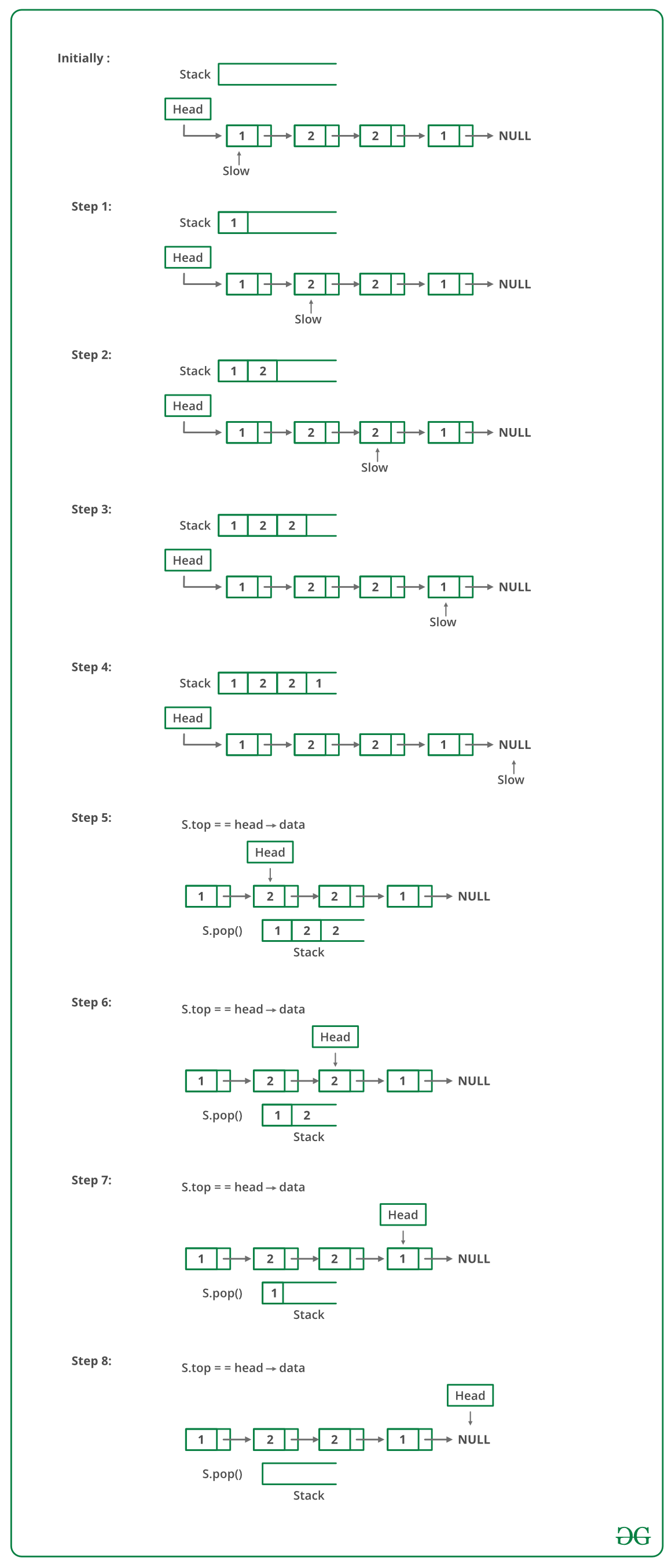GeeksforGeeks App
Open AppBrowser
Continue

# Python Program To Check If A Singly Linked List Is Palindrome

Given a singly linked list of characters, write a function that returns true if the given list is a palindrome, else false.METHOD 1 (Use a Stack):

• A simple solution is to use a stack of list nodes. This mainly involves three steps.
• Traverse the given list from head to tail and push every visited node to stack.
• Traverse the list again. For every visited node, pop a node from the stack and compare data of popped node with the currently visited node.
• If all nodes matched, then return true, else false.

Below image is a dry run of the above approach:Below is the implementation of the above approach :

## Python3

 `# Python3 program to check if linked``# list is palindrome using stack``class` `Node:``    ``def` `__init__(``self``, data):       ``        ``self``.data ``=` `data``        ``self``.ptr ``=` `None``        ` `# Function to check if the linked list``# is palindrome or not``def` `ispalindrome(head):``    ` `    ``# Temp pointer``    ``slow ``=` `head` `    ``# Declare a stack``    ``stack ``=` `[]``    ` `    ``ispalin ``=` `True` `    ``# Push all elements of the list``    ``# to the stack``    ``while` `slow !``=` `None``:``        ``stack.append(slow.data)``        ` `        ``# Move ahead``        ``slow ``=` `slow.ptr` `    ``# Iterate in the list again and``    ``# check by popping from the stack``    ``while` `head !``=` `None``:` `        ``# Get the top most element``        ``i ``=` `stack.pop()``        ` `        ``# Check if data is not``        ``# same as popped element``        ``if` `head.data ``=``=` `i:``            ``ispalin ``=` `True``        ``else``:``            ``ispalin ``=` `False``            ``break` `        ``# Move ahead``        ``head ``=` `head.ptr``        ` `    ``return` `ispalin` `# Driver Code` `# Addition of linked list``one ``=` `Node(``1``)``two ``=` `Node(``2``)``three ``=` `Node(``3``)``four ``=` `Node(``4``)``five ``=` `Node(``3``)``six ``=` `Node(``2``)``seven ``=` `Node(``1``)` `# Initialize the next pointer``# of every current pointer``one.ptr ``=` `two``two.ptr ``=` `three``three.ptr ``=` `four``four.ptr ``=` `five``five.ptr ``=` `six``six.ptr ``=` `seven``seven.ptr ``=` `None` `# Call function to check``# palindrome or not``result ``=` `ispalindrome(one)` `print``(``"isPalindrome:"``, result)` `# This code is contributed by Nishtha Goel`

Output:

`isPalindrome: true`

Time complexity: O(n), where n represents the length of the given linked list.

Auxiliary Space: O(n), for using a stack, where n represents the length of the given linked list.

METHOD 2 (By reversing the list):
This method takes O(n) time and O(1) extra space.
1) Get the middle of the linked list.
2) Reverse the second half of the linked list.
3) Check if the first half and second half are identical.
4) Construct the original linked list by reversing the second half again and attaching it back to the first half

To divide the list into two halves, method 2 of this post is used.

When a number of nodes are even, the first and second half contain exactly half nodes. The challenging thing in this method is to handle the case when the number of nodes is odd. We don’t want the middle node as part of the lists as we are going to compare them for equality. For odd cases, we use a separate variable ‘midnode’.

## Python3

 `# Python program to check if``# linked list is palindrome` `# Node class``class` `Node:` `    ``# Constructor to initialize``    ``# the node object``    ``def` `__init__(``self``, data):       ``        ``self``.data ``=` `data``        ``self``.``next` `=` `None` `class` `LinkedList:` `    ``# Function to initialize head``    ``def` `__init__(``self``):       ``        ``self``.head ``=` `None` `    ``# Function to check if given``    ``# linked list is palindrome or not``    ``def` `isPalindrome(``self``, head):       ``        ``slow_ptr ``=` `head``        ``fast_ptr ``=` `head``        ``prev_of_slow_ptr ``=` `head``        ` `        ``# To handle odd size list``        ``midnode ``=` `None``        ` `        ``# Initialize result``        ``res ``=` `True` `        ` `        ``if` `(head !``=` `None` `and``            ``head.``next` `!``=` `None``):``            ` `            ``# Get the middle of the list.``            ``# Move slow_ptr by 1 and``            ``# fast_ptrr by 2, slow_ptr``            ``# will have the middle node``            ``while` `(fast_ptr !``=` `None` `and``                   ``fast_ptr.``next` `!``=` `None``):``                      ` `                ``# We need previous of the slow_ptr``                ``# for linked lists  with odd``                ``# elements``                ``fast_ptr ``=` `fast_ptr.``next``.``next``                ``prev_of_slow_ptr ``=` `slow_ptr``                ``slow_ptr ``=` `slow_ptr.``next``                ` `            ``# fast_ptr would become NULL when``            ``# there are even elements in the``            ``# list and not NULL for odd elements.``            ``# We need to skip the middle node for``            ``# odd case and store it somewhere so``            ``# that we can restore the original list``            ``if` `(fast_ptr !``=` `None``):``                ``midnode ``=` `slow_ptr``                ``slow_ptr ``=` `slow_ptr.``next``                ` `            ``# Now reverse the second half``            ``# and compare it with the first half``            ``second_half ``=` `slow_ptr``            ` `            ``# NULL terminate first half``            ``prev_of_slow_ptr.``next` `=` `None``            ` `            ``# Reverse the second half``            ``second_half ``=` `self``.reverse(second_half)``            ` `            ``# Compare``            ``res ``=` `self``.compareLists(head, second_half) ``            ` `            ``# Construct the original list back``            ``# Reverse the second half again``            ``second_half ``=` `self``.reverse(second_half)``            ` `            ``if` `(midnode !``=` `None``):``                ` `                ``# If there was a mid node (odd size``                ``# case) which was not part of either``                ``# first half or second half.``                ``prev_of_slow_ptr.``next` `=` `midnode``                ``midnode.``next` `=` `second_half``            ``else``:``                ``prev_of_slow_ptr.``next` `=` `second_half``        ``return` `res``    ` `    ``# Function to reverse the linked list``    ``# Note that this function may change``    ``# the head``    ``def` `reverse(``self``, second_half):``        ` `        ``prev ``=` `None``        ``current ``=` `second_half``        ``next` `=` `None``        ` `        ``while` `current !``=` `None``:``            ``next` `=` `current.``next``            ``current.``next` `=` `prev``            ``prev ``=` `current``            ``current ``=` `next``            ` `        ``second_half ``=` `prev``        ``return` `second_half` `    ``# Function to check if two input``    ``# lists have same data``    ``def` `compareLists(``self``, head1, head2):``        ` `        ``temp1 ``=` `head1``        ``temp2 ``=` `head2``        ` `        ``while` `(temp1 ``and` `temp2):``            ``if` `(temp1.data ``=``=` `temp2.data):``                ``temp1 ``=` `temp1.``next``                ``temp2 ``=` `temp2.``next``            ``else``:``                ``return` `0``                ` `        ``# Both are empty return 1``        ``if` `(temp1 ``=``=` `None` `and` `temp2 ``=``=` `None``):``            ``return` `1``            ` `        ``# Will reach here when one is NULL``        ``# and other is not``        ``return` `0``    ` `    ``# Function to insert a new node``    ``# at the beginning``    ``def` `push(``self``, new_data):``        ` `        ``# Allocate the Node &``        ``# Put in the data``        ``new_node ``=` `Node(new_data)``        ` `        ``# Link the old list of the new one``        ``new_node.``next` `=` `self``.head``        ` `        ``# Move the head to point to the``        ``# new Node``        ``self``.head ``=` `new_node` `    ``# A utility function to print``    ``# a given linked list``    ``def` `printList(``self``):``        ` `        ``temp ``=` `self``.head``        ` `        ``while``(temp):``            ``print``(temp.data, end ``=` `"->"``)``            ``temp ``=` `temp.``next``            ` `        ``print``(``"NULL"``)` `# Driver code``if` `__name__ ``=``=` `'__main__'``:   ``    ``l ``=` `LinkedList()``    ``s ``=` `[``'a'``, ``'b'``, ``'a'``,``         ``'c'``, ``'a'``, ``'b'``, ``'a'``]``    ` `    ``for` `i ``in` `range``(``7``):``        ``l.push(s[i])``        ``l.printList()``        ` `        ``if` `(l.isPalindrome(l.head) !``=` `False``):``            ``print``(``"Is Palindrome"``)``        ``else``:``            ``print``(``"Not Palindrome"``)``        ``print``()` `# This code is contributed by MuskanKalra1`

Output:

```a->NULL
Is Palindrome

b->a->NULL
Not Palindrome

a->b->a->NULL
Is Palindrome

c->a->b->a->NULL
Not Palindrome

a->c->a->b->a->NULL
Not Palindrome

b->a->c->a->b->a->NULL
Not Palindrome

a->b->a->c->a->b->a->NULL
Is Palindrome```

Time Complexity: O(n)
Auxiliary Space: O(1)

Please refer complete article on Function to check if a singly linked list is palindrome for more details!

My Personal Notes arrow_drop_up